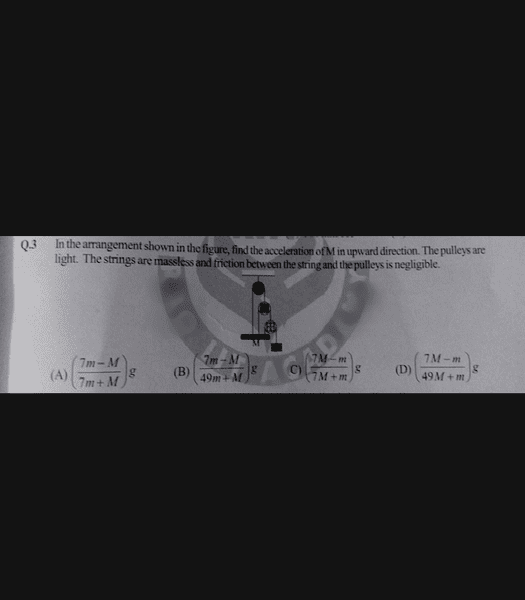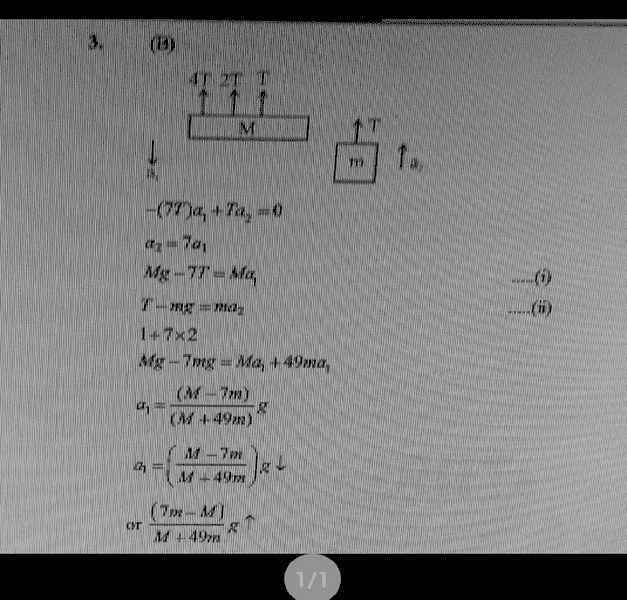# Newton's laws problem?

In the arrangement shown in the figure, find the acceleration of M in upward direction. The pulleys are light. The strings are mass less and friction between the string and the pulleys is negligible.The options are given. The final answer is (B) and this was the solution:I don't understand the 1+7x2 step right there.
Also I fail to understand why there is 49m in the denominator of the solution instead of 7m (I'm assuming the equation is multiplied by 7. I might be wrong though.)
Can anyone explain me the solutions please? Thanks.

P.S.: Sorry about the low quality images, it was in a kind of jiffy.

mfb
Mentor
The solution makes more sense without the line "1+7*2". The line above it gets multiplied by 7 and then plugged into the equation above it.

I don't understand the purpose of '1+7x2' either...

The solution makes more sense without the line "1+7*2". The line above it gets multiplied by 7 and then plugged into the equation above it.

Even if equation (ii) multiplied by 7, the equation must be something like this: 7T - 7mg = 7ma2
So, on adding the two equations, 7T and -7T will get cancelled out and the result will be Mg-7mg = Ma1 + 7ma1 ...(the acceleration is equal. therefore a1 = a2 .)
I don't understand where the 49ma1 comes from.

mfb
Mentor
The accelerations are not equal.

The accelerations are not equal.
Then how come they changed ma2 to ma1 in the fourth last step?
That way, the step should be written as Mg-7mg = Ma1 + 7ma2 right?

mfb
Mentor
Then how come they changed ma2 to ma1 in the fourth last step?
They didn't, those are two different equations.
That way, the step should be written as Mg-7mg = Ma1 + 7ma2 right?
That is a possible way to write it.

Note the first two lines in the solution.

•MARK 42
That is a possible way to write it.

Note the first two lines in the solution.

Oh, got it. Sorry, silly mistakes. Thanks you were really helpful.